Distribution Analyses

## Empirical CDF

The empirical distribution function of a sample, Fn(y), is the proportion of observations less than or equal to y.
where n is the number of observations, and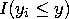is an indicator function with value 1 if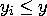and with value 0 otherwise.

The Kolmogorov statistic D is a measure of the discrepancy between the empirical distribution and the hypothesized distribution.
where F(y) is the hypothesized cumulative distribution function. The statistic is the maximum vertical distance between the two distribution functions. The Kolmogorov statistic can be used to construct a confidence band for the unknown distribution function, to test for a hypothesized completely known distribution, and to test for a specific family of distributions with unknown parameters.

If you select a Weight variable, the weighted empirical distribution function is the proportion of observation weights for observations less than or equal to y.
Copyright © 2007 by SAS Institute Inc., Cary, NC, USA. All rights reserved.